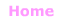Aa Bb Cc Dd Ee Ff Gg Hh Ii Jj Kk Ll Mm Nn Oo Pp Qq Rr Ss Tt Uu Vv Ww Xx Yy Zz

Ff
face
fact family
factor, factor pair
factor tree
factorial
factorise
Fahrenheit
feet, foot
few
Fibonacci numbers
figurate numbers
finite
first
first quartile
five, fifteen, fifty
five frames
five number summary
flat
flip
fluid ounce
FOIL
formula
fortnight

four, fourteen, forty
fraction
frequency
frequency distribution table
frequency histogram
frequency polygon
front view
frustum
function
fundamental theorem of arithmetic© Jenny Eather 2014. All rights reserved.